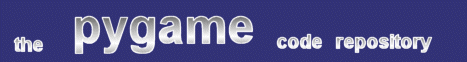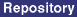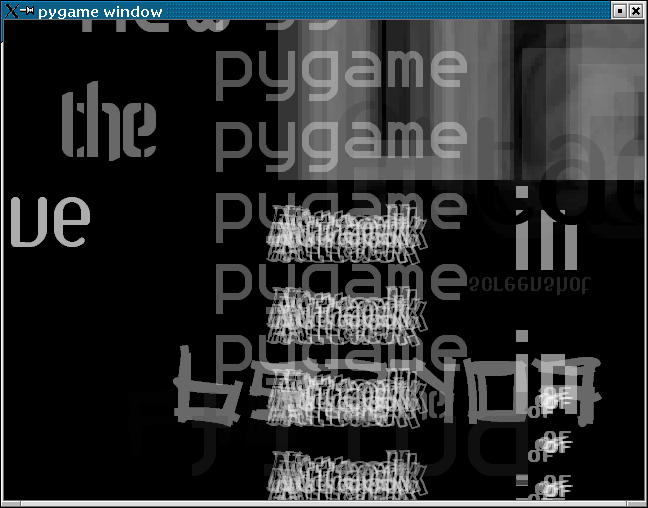### Title: Skyscraper

Author: Richard Jones (richardjones at optushome.com.au)
Submission date: December 09, 2001

Description: Displays words loaded from the supplied website, using a variety of typographic effects.pygame version required: Any (Numeric Required)
SDL version required: Any
Python version required: Any

Comments: I haven't read the book Mr. Jones refers to, although I've seen the Underworld album cover, and the effect looks right. Invoke the program like this :

`python skyscraper.py url`
, where url is something like "http://www.pygame.org". The script is sensitive to the presence of fonts on your system - if you start getting errors, try putting a couple of fonts in the script's directory.

```
#!/usr/bin/env python
'''mmm...
skyscraper       i love you

this program is inspired by the book "mmm... skyscraper i love you" by
Tomato / Underworld

i would like to try to set it to music some day

it would also be nice to have some more structure to the chaos... do some
low-frequency stuff first and then a couple of high-frequency bits...

richardjones@optushome.com.au

thanks to pete shinners for pygame and the original font demo
graham for the fonts... http://grudnuk.com/fonts/

this code is placed in the public domain
'''

# import every damn thing we can find...
import os, sys, math, random, time
import urllib, htmllib, formatter, string, re
try:
import pygame
except ImportError, message:
print "You need pygame to run this script! (%s)"%message
sys.exit(0)
import pygame.font
import pygame.image
import pygame.transform
import pygame.surfarray
from pygame.locals import *
try:
from Numeric import array
except ImportError, message:
print "You need Numeric to run this script! (%s)"%message
sys.exit(0)

class Skyscraper:
# initialise the fonts array
data = 'data'
fonts = []
for entry in os.listdir(data):
fonts.append(os.path.join(data, entry))

def __init__(self, text, screensize):
'''Generate an image using the text (a single word) using a random
font, size and intensity. Then manipulate it randomly using any of
the do_foo methods.
'''
self.screensize = screensize
size = random.randint(1, 200)
face = random.choice(self.fonts)
font = pygame.font.Font(face, size)
level = random.randint(0, 255)
surf = font.render(text, 2, (level, level, level))
self.surface = surf
for i in range(random.randint(1, 2)):
method = random.choice(self.methods)
meth = getattr(self, method)()

def do_subtract(self):
'''Invert the image so it'll subtract from the destination.
This is achieved by just setting the colour to black and leaving
the alpha channel alone.
'''
s = pygame.surfarray.pixels3d(self.surface)
s[:,:,:] = 0

def do_nothing(self):
'''Leave the text alone, just like it was when the stork dropped it
off.
'''
pass

def do_smudge(self):
'''Jiggle the text around a bit.
We fiddle the image's alpha channel and then repeatedly draw it
onto another surface at different positions.
'''
src = self.surface
w, h = src.get_size()
# make the width a little saner so we don't do unnecessary
# processing
if w > self.screensize:
w = self.screensize

# halve the alpha channel
a = pygame.surfarray.pixels_alpha(src)
a /= array(random.randint(1, 8)).astype('b')
newsize = w+20, h+20
temp = pygame.Surface(newsize, 0, 24)
for i in range(random.randint(5, 10)):
temp.blit(src, (random.randint(0, 20), random.randint(0, 20)))

# make a new surface with alhpa and copy in the red channel from
# the greyscale temp surface as the alpha channel.
self.surface = pygame.Surface(newsize, 0, src)
self.surface.fill((255,255,255,255))
newalpha = pygame.surfarray.pixels_alpha(self.surface)
srcalpha = pygame.surfarray.pixels3d(temp)
newalpha[:,:] = srcalpha[:,:,0]

def do_repeat(self):
'''Repeat the text on 2 <= n < =20 lines.
'''
src = self.surface
w, h = src.get_size()
if w > self.screensize:
w = self.screensize

# same as smudge, but we halve the alpha channel
n = random.randint(2, 20)
max_n = self.screensize/h
if n > max_n: n = max_n
newsize = w, h * n
temp = pygame.Surface(newsize, 0, 24)
for i in range(n):
temp.blit(src, (0, h*i))

# make a new surface with alhpa and copy in the red channel from
# the greyscale temp surface as the alpha channel.
self.surface = pygame.Surface(newsize, 0, src)
self.surface.fill((255,255,255,255))
newalpha = pygame.surfarray.pixels_alpha(self.surface)
srcalpha = pygame.surfarray.pixels3d(temp)
newalpha[:,:] = srcalpha[:,:,0]

def do_mag(self):
'''Do a simple magnification of the text.
'''
src = self.surface
w, h = src.get_size()
# figure the size of subsection of the surface
sw, sh = w/2, h/2
x, y = random.randint(0, w-sw), random.randint(0, h-sh)
dst = src.subsurface((x, y, sw, sh))
self.surface = pygame.transform.scale(dst, (w*2, h*2))

def do_flip(self):
'''Flip the text in one or more axes.
'''
a = pygame.surfarray.pixels_alpha(self.surface)
a /= array(2).astype('b')
self.surface = pygame.transform.flip(self.surface,
random.randint(0,1), random.randint(0,1))

class WordSucker(formatter.NullWriter):
'''This is a writer for the parser/formatter/writer trio that does
HTMLParsing in python. All I do here is accept the data (which will
be the stuff between HTML tags) and accept all the words - which I
define as only having letters in them - no numbers, no digits, ...
'''
def __init__(self):
formatter.NullWriter.__init__(self)
self.words = []

def send_flowing_data(self, data):
for word in data.split():
if re.search(r'[\W\d_]', word): continue
self.words.append(word)

def main():
# figure all the fun methods
Skyscraper.methods = []
for method in Skyscraper.__dict__.keys():
if method.startswith('do_'):
Skyscraper.methods.append(method)

pygame.init()

# create a window the correct size
width, height = 640, 480
win = pygame.display.set_mode((width, height)) #, FULLSCREEN)

#wait for the finish
finished = 0

# get the words!
if len(sys.argv) > 1:
f = urllib.urlopen(sys.argv)
else:
f = sys.stdin
if f is not sys.stdin:
f.close()
wordsucker = WordSucker()
parser = htmllib.HTMLParser(formatter.AbstractFormatter(wordsucker))
parser.feed(data)
parser.close()

# loop indefinitely, displaying all the words we got from the source.
# Clear the display once in a while
i = 0
target = random.randint(10,20)
while 1:
for entry in wordsucker.words:
for event in pygame.event.get():
if event.type is KEYDOWN and event.key == K_s: #save it
name = os.path.splitext(sys.argv) + '.bmp'
print 'Saving image to:', name
pygame.image.save(win, name)
elif event.type in (QUIT, KEYDOWN, MOUSEBUTTONDOWN):
sys.exit(0)

# delay and possibly clear
if i > target:
time.sleep(5)
win.fill((0,0,0))
i = 0
target = random.randint(10,20)
i = i + 1

# create one of our random images
im = Skyscraper(entry, (width, height))
w, h = im.surface.get_size()
x, y = random.randint(-w, width), random.randint(-h, height)
win.blit(im.surface, (x, y))
pygame.display.flip()

if __name__ == '__main__':
if sys.argv == '-h':
print "Usage: %s <url to load text from>"
else:
main()

```
 From: Anonymous Date: January 04, 2003 09:56 GMT ineed a grear and modern skyscraper plan

 Feedback